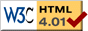# Inverted Vibrating Pendulum

previous next
• Sim
• Graph
• Time Graph
• Multi Graph

Physics-based simulation of a vibrating pendulum with a pivot point is shaking rapidly up and down. Surprisingly, the position with the pendulum being vertically upright is stable, so this is also known as the inverted pendulum.

Click and drag near the pendulum to modify its position. The anchor can also be moved. Enable the "show controls" checkbox to set gravity, frequency of oscillation, magnitude of oscillation, and damping (friction).

A regular non-vibrating pendulum is stable only when it is hanging straight down. In this simulation, the support pivot point of the pendulum is oscillating rapidly up and down. When the oscillation is rapid and of small amplitude, there is a second stable position, with the pendulum standing straight up, in a vertical upright "upside-down" position.

You can build a physical version of the inverted pendulum using a jigsaw to power the oscillations, see references below. This is a popular demonstration used in university physics or math classes.

Experiments to try:

• Disturb the pendulum from its stable inverted position. Can it recover? How far can you disturb it?
• What is the slowest frequency that still has the stable inverted position?
• What is the range of amplitude that still has the stable inverted position?
• For a faster or slower frequency, does the range of amplitude change?
• Does the strength of gravity affect whether the inverted pendulum is stable?
• How does friction (damping) affect the inverted pendulum?
• Make the time step smaller to get more calculations per second, which will give a smoother graph.

The time rate adjustment makes the simulation run slower than real-time so that smaller time steps are used, which gives better accuracy and smoother graph plots. The physics and math is all the same, it is only displayed at a slower rate than it would be in real time.

The mathematics of this simulation is given at the Moveable Pendulum web page. Also available are: open source code, documentation and a simple-compiled version which is more customizable.

There are actually two simultaneous simulations happening here: the pendulum, and the anchor block. The anchor block is modelled as a point mass and can be moved by a force such as that applied when dragging with the mouse near the anchor point. The anchor block is not affected by the pendulum. See Anchor Block Dynamics on the Moveable Pendulum page for the math. The oscillatory motion of the anchor is caused by applying a time varying force to the anchor point. This means that you can also drag the anchor point with your mouse, which applies another force. An alternative method would be specify where the anchor point is in space at any given time with an equation, so that the anchor point could not move in any way other than this equation of oscillation.

## References

previous next